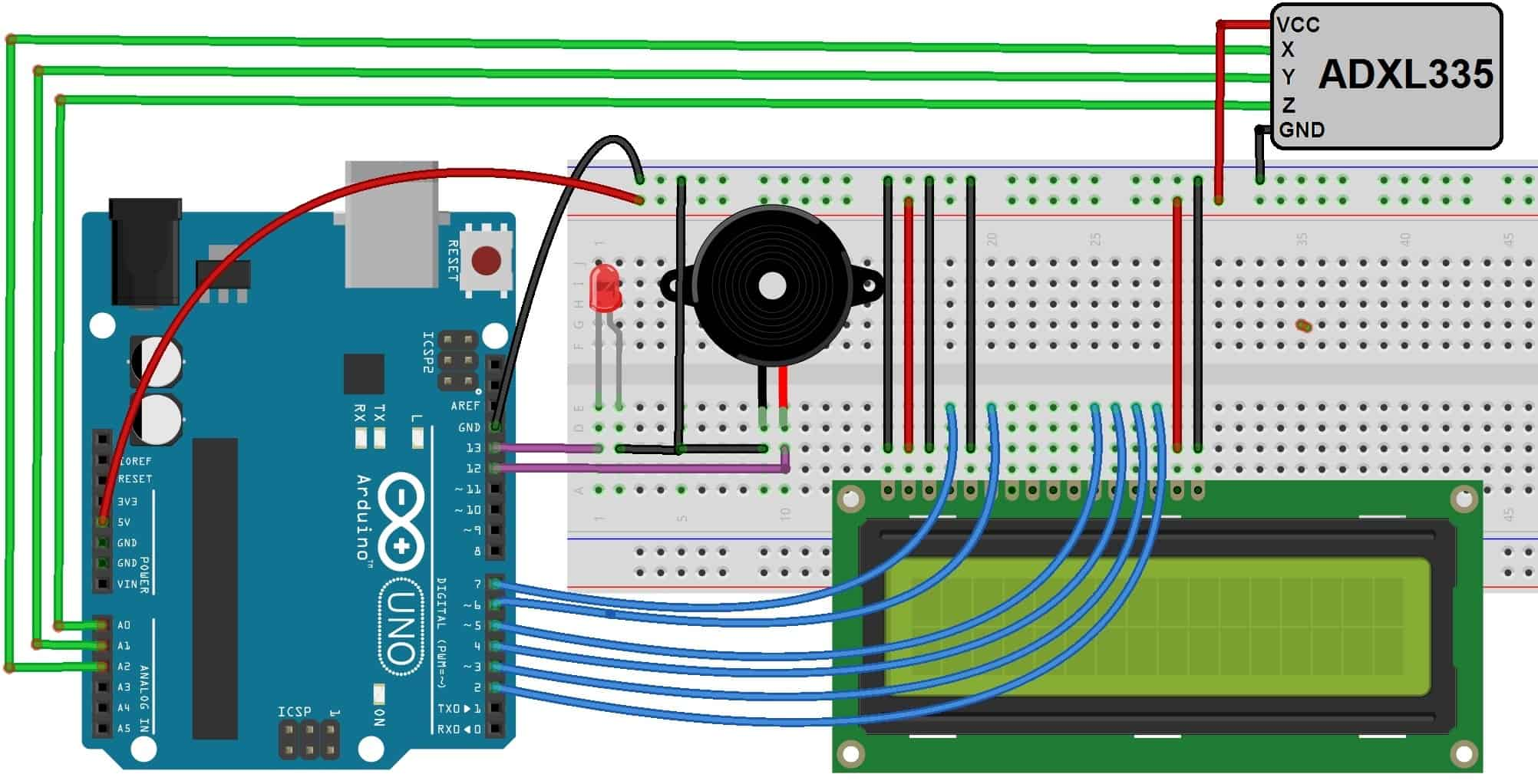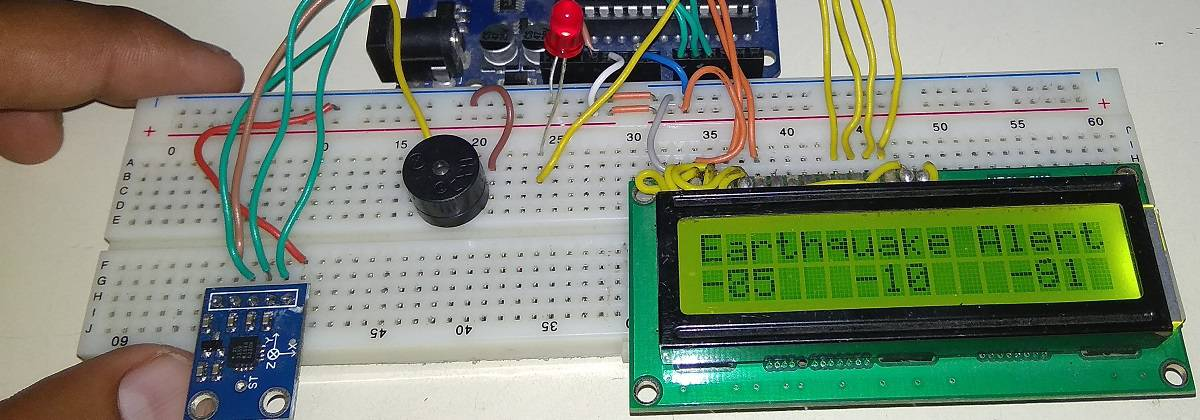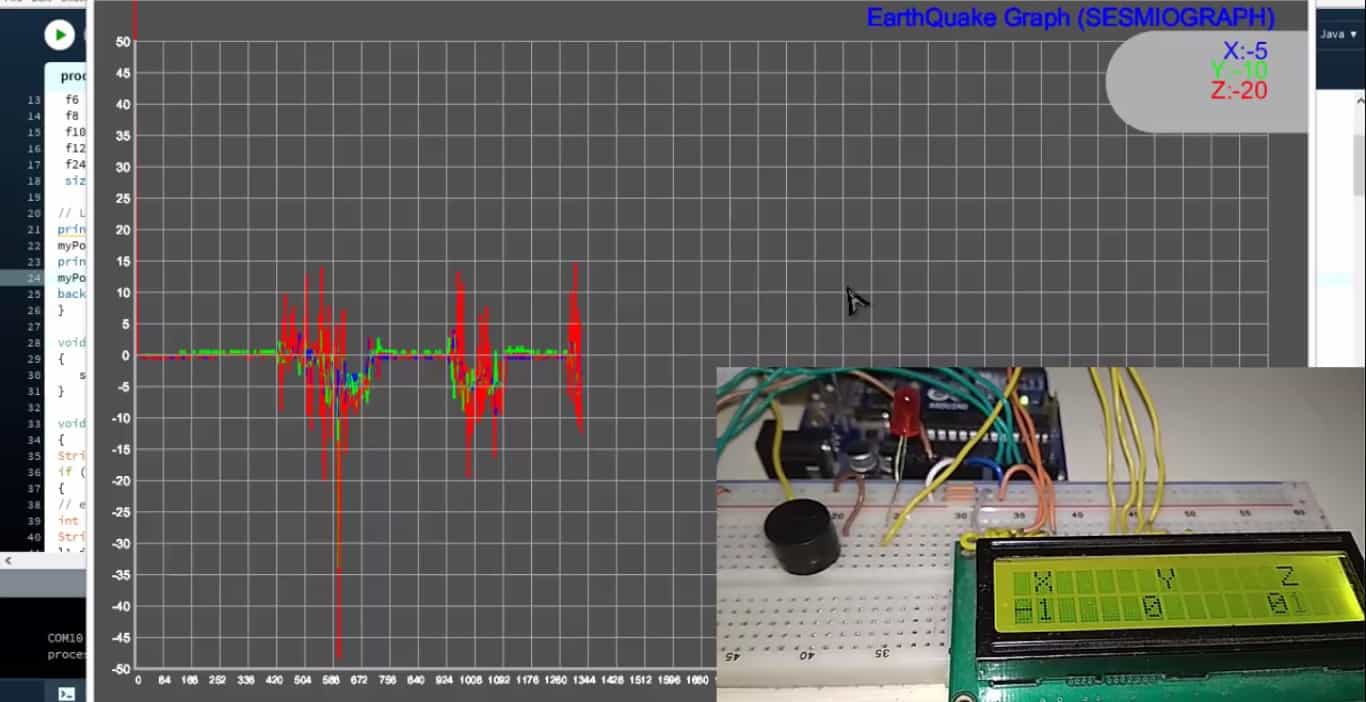# Arduino Earthquake Detector Alarm with Seismic Graph using Accelerometer(Last Updated On: February 25, 2019)

## Introduction:

In this project we will learn how to design Arduino Earthquake Detector Alarm with Seismic Graph. We have used ADXL335 3 axis Accelerometer as a sensor for detecting tilting, trembling or any shaking movement of earthquake. We have interfaced ADXL335 Accelerometer with Arduino and LCD display for designing Arduino Earthquake Detector Alarm with Seismic Graph.

To learn detail about Accelerometer Working and Tutorial you can visit:
1. Accelerometer Basics and Tutorial Explanation
2. Acceleration Measurement with Accelerometer ADXL335 & Arduino

The Arduino code as well as procesing IDE code both are given below. The processing IDE code helps in drawing the graph of the tilting state. The buzzer or LED is used as an alarm whenever the shaking threshold goes higher.

## ADXL335 3 Axis Accelerometer:

### Introduction:This Accelerometer module is based on the popular ADXL335 three-axis analog accelerometer IC, which reads off the X, Y and Z acceleration as analog voltages. By measuring the amount of acceleration due to gravity, an accelerometer can figure out the angle it is tilted at with respect to the earth. By sensing the amount of dynamic acceleration, the accelerometer can find out how fast and in what direction the device is moving. Using these two properties, you can make all sorts of cool projects, from musical instruments (imagine playing and having the tilt connected to the distortion level or the pitch-bend) to a velocity monitor on your car (or your children’s car). The accelerometer is very easy interface to an Arduino Micro-controller using 3 analog input pins, and can be used with most other micro controllers, such as the PIC or AVR.

### Features:

```1. 3V-6V DC Supply Voltage
2. Onboard LDO Voltage regulator
3. Can be interface with 3V3 or 5V Microcontroller.
4. All necessary Components are populated.
5. Ultra Low Power: 40uA in measurement mode, 0.1uA in [email protected] 2.5V
6. Tap/Double Tap Detection
7. Free-Fall Detection
8. Analog output```

### Working of ADXL335 Accelerometer:

The most commonly used device is the piezoelectric accelerometer. As the name suggests, it uses the principle of piezoelectric effect. The device consists of a piezoelectric quartz crystal on which an accelerative force, whose value is to be measured, is applied.Due to the special self-generating property, the crystal produces a voltage that is proportional to the accelerative force. The working and the basic arrangement is shown in the figure below.

## Arduino Earthquake Detector Alarm with Seismic Graph using Accelerometer:

### Components Required:

```1. Arduino Uno Board
2. ADXL335 Accelerometer - (Buy Online from Amazon)
3. 16 x 2 LCD Display
4. Buzzer
5. LED```

### Circuit Diagram & Connections:## Working Explanation:

In this Arduino Earthquake Detector Alarm with Seismic Graph project, we have made two codes: one for Arduino to detect an earthquake and another for Processing IDE to plot the earthquake vibrations over the graph on Computer.

Download the processing IDE from here: Processing IDE Link

First upload the Arduino Code/program to Arduino UNO board. After that open processing IDE. Copy the code from below and paste it on IDE. Then the next step is hit on run. As soon as you hit run the graph starts running. So shake the accelerometer and observe the graph.## Arduino Source Code/Program:

```#include<LiquidCrystal.h> // lcd Header
LiquidCrystal lcd(7,6,5,4,3,2); // pins for LCD Connection

#define buzzer 12 // buzzer pin
#define led 13 //led pin

#define x A0 // x_out pin of Accelerometer
#define y A1 // y_out pin of Accelerometer
#define z A2 // z_out pin of Accelerometer

/*variables*/
int xsample=0;
int ysample=0;
int zsample=0;
long start;
int buz=0;

/*Macros*/
#define samples 50
#define maxVal 20 // max change limit
#define minVal -20 // min change limit
#define buzTime 5000 // buzzer on time

void setup()
{
lcd.begin(16,2); //initializing lcd
Serial.begin(9600); // initializing serial
delay(1000);
lcd.print("EarthQuake ");
lcd.setCursor(0,1);
lcd.print("Detector ");
delay(2000);
lcd.clear();
lcd.print("Calibrating.....");
lcd.setCursor(0,1);
lcd.print("Please wait...");
pinMode(buzzer, OUTPUT);
pinMode(led, OUTPUT);
buz=0;
digitalWrite(buzzer, buz);
digitalWrite(led, buz);
for(int i=0;i<samples;i++) // taking samples for calibration
{
xsample+=analogRead(x);
ysample+=analogRead(y);
zsample+=analogRead(z);
}

xsample/=samples; // taking avg for x
ysample/=samples; // taking avg for y
zsample/=samples; // taking avg for z

delay(3000);
lcd.clear();
lcd.print("Calibrated");
delay(1000);
lcd.clear();
lcd.print("Device Ready");
delay(1000);
lcd.clear();
lcd.print(" X Y Z ");
}

void loop()
{
int value1=analogRead(x); // reading x out
int value2=analogRead(y); //reading y out
int value3=analogRead(z); //reading z out

int xValue=xsample-value1; // finding change in x
int yValue=ysample-value2; // finding change in y
int zValue=zsample-value3; // finding change in z

/*displying change in x,y and z axis values over lcd*/
lcd.setCursor(0,1);
lcd.print(xValue);
lcd.setCursor(6,1);
lcd.print(yValue);
lcd.setCursor(12,1);
lcd.print(zValue);
delay(100);

/* comparing change with predefined limits*/
if(xValue < minVal || xValue > maxVal || yValue < minVal || yValue > maxVal || zValue < minVal || zValue > maxVal)
{
if(buz == 0)
start=millis(); // timer start
buz=1; // buzzer / led flag activated
}

else if(buz == 1) // buzzer flag activated then alerting earthquake
{
lcd.setCursor(0,0);
lcd.print("Earthquake Alert ");
if(millis()>= start+buzTime)
buz=0;
}

else
{
lcd.clear();
lcd.print(" X Y Z ");
}

digitalWrite(buzzer, buz); // buzzer on and off command
digitalWrite(led, buz); // led on and off command

/*sending values to processing for plot over the graph*/
Serial.print("x=");
Serial.println(xValue);
Serial.print("y=");
Serial.println(yValue);
Serial.print("z=");
Serial.println(zValue);
Serial.println(" \$");
}```

## Processing IDE Code/Program:

```import processing.serial.*;
PFont f6,f8,f12,f10;
PFont f24;
Serial myPort; // The serial port
int xPos = 0; // horizontal position of the graph
float y1=0;
float y2=0;
float y3=0;

void setup ()
{
// set the window size: and Font size
f6 = createFont("Arial",6,true);
f8 = createFont("Arial",8,true);
f10 = createFont("Arial",10,true);
f12 = createFont("Arial",12,true);
f24 = createFont("Arial",24,true);
size(1200, 700);

// List all the available serial ports
println(Serial.list());
myPort = new Serial(this, "COM10", 9600);
println(myPort);
myPort.bufferUntil('\n');
background(80);
}

void draw ()
{
serial ();
}

void serial()
{
String inString = myPort.readStringUntil('\$'); // reading incomming date from serial
if (inString != null)
{
// extracting all required values of all three axis:
int l1=inString.indexOf("x=")+2;
String temp1=inString.substring(l1,l1+3);
l1=inString.indexOf("y=")+2;
String temp2=inString.substring(l1,l1+3);
l1=inString.indexOf("z=")+2;
String temp3=inString.substring(l1,l1+3);

//mapping x, y and z value with graph dimensions
float inByte1 = float(temp1+(char)9);
inByte1 = map(inByte1, -80,80, 0, height-80);
float inByte2 = float(temp2+(char)9);
inByte2 = map(inByte2,-80,80, 0, height-80);
float inByte3 = float(temp3+(char)9);
inByte3 = map(inByte3,-80,80, 0, height-80);
float x=map(xPos,0,1120,40,width-40);

//ploting graph window, unit
strokeWeight(2);
stroke(175);
Line(0,0,0,100);
textFont(f24);
fill(0,00,255);
textAlign(RIGHT);
xmargin("EarthQuake Graph (SESMIOGRAPH)",200,100);

fill(100);
strokeWeight(100);
line(1050,80,1200,80);

strokeWeight(1);
textAlign(RIGHT);
fill(0,0,255);
String temp="X:"+temp1;
Text(temp,100,95);

fill(0,255,0);
temp="Y:"+temp2;
Text(temp,100,92);

fill(255,0,0);;
temp="Z:"+temp3;
Text(temp,100,89);

//ploting x y and z values over graph
strokeWeight(2);
int shift=40;

stroke(0,0,255);
if(y1 == 0)
y1=height-inByte1-shift;
line(x, y1, x+2, height-inByte1-shift) ;
y1=height-inByte1-shift;

stroke(0,255,0);
if(y2 == 0)
y2=height-inByte2-shift;
line(x, y2, x+2, height-inByte2-shift) ;
y2=height-inByte2-shift;

stroke(255,0,0);
if(y2 == 0)
y3=height-inByte3-shift;
line(x, y3, x+2, height-inByte3-shift) ;
y3=height-inByte3-shift;

xPos+=1;

if (x >= width-30) // go back to begining
{
xPos = 0;
background(80);
}
}
}

void Line(int x1, int y1, int x2, int y2)
{
float xx1=map(x1,0,100,40,width-40);
float xx2=map(x2,0,100,40,width-40);
float yy1=map(y1,0,100,height-40,40);
float yy2=map(y2,0,100,height-40,40);

line(xx1,yy1,xx2,yy2);
xx2=map(100,0,100,40,width-40);
yy2=map(0,0,100,height-40,40);
line(xx1,yy1,xx2,yy2);

strokeWeight(1);
for(int i=1;i<21;i++)
{
yy2=map(i*10,0,200,height-40,40);
yy1=yy2;
line(xx1,yy1,xx2,yy2);
}
yy2=map(100,0,100,height-40,40);
yy1=map(0,0,100,height-40,40);
for(int i=1;i<41;i++)
{
xx1=map(i*5,0,200,40,width-40);
xx2=map(i*5,0,200,40,width-40);
line(xx1,yy1,xx2,yy2);
}

textAlign(RIGHT); // 100 degree
// result+=yy1;
fill(255);
strokeWeight(1);
textFont(f12);
for(int i=-10;i<11;i++)
{
String result="";
result+=5*i;
ymargin(result, x1,y1);
y1+=5;
}

x1=0;
y1=0;
strokeWeight(1);
textFont(f10);
for(int i=0;i<41;i++)
{
String result="";
result+=28*3*i;
xmargin(result, x1,y1);
x1+=5;
}

textAlign(RIGHT);

textAlign(RIGHT);
}

void ymargin(String value, int x1, int y1)
{

float xx1=map(x1,0,100,40,width-40);
float yy1=map(y1,0,100,height-40,40);
text(value,xx1-5,yy1+5);
}

void xmargin(String value, int x1, int y1)
{

float xx1=map(x1,0,200,40,width-40);
float yy1=map(y1,0,100,height-25,25);
text(value,xx1+7,yy1);
}

void Text(String value, int x1, int y1)
{

float xx1=map(x1,0,100,40,width-40);
float yy1=map(y1,0,100,height-25,25);
text(value,xx1,yy1);
}```

## 3 Comments

1.janelle says:

Does it work all day long?

1.Alex Newton says:

It will work for several years too

2.Mischelle Labe says:

Can I ask what is wrong if after shaking the accelerometer then placing it t rest, the buzzer won’t stop